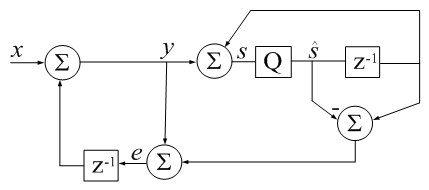# Accumulator Error Feedback

(Difference between revisions)
 Revision as of 23:35, 28 November 2017 (edit)← Previous diff Revision as of 23:45, 28 November 2017 (edit) (undo)Next diff → Line 1: Line 1: [[Image:Gleich.jpg|thumb|right|429px|CSUM() in Digital Signal Processing terms: [[Image:Gleich.jpg|thumb|right|429px|CSUM() in Digital Signal Processing terms: - z-1 is a unit delay, Q a floating-point quantizer to 64 bits, + z-1 is a unit delay, Q a 64-bit floating-point quantizer, - qi represents error due to quantization (additive by definition). + qi represents error due to quantization (additive by definition). Algebra represents neither a sequence of instructions or algorithm. Algebra represents neither a sequence of instructions or algorithm. It is only meant to remind that an imperfect accumulator introduces noise into a series.]] It is only meant to remind that an imperfect accumulator introduces noise into a series.]] Line 50: Line 50: should begin the csum() subroutine to achieve the most accurate summation. should begin the csum() subroutine to achieve the most accurate summation. That is not presented here because the commented Example (inspired by Higham) would then display false positive results. That is not presented here because the commented Example (inspired by Higham) would then display false positive results. - Even in absence of sorting, csum() is more accurate than conventional summation by orders of magnitude. + Even in complete absence of sorting, csum() can be more accurate than conventional summation by orders of magnitude. === links === === links ===

## Revision as of 23:45, 28 November 2017CSUM() in Digital Signal Processing terms: z-1 is a unit delay, Q a 64-bit floating-point quantizer, qi represents error due to quantization (additive by definition). Algebra represents neither a sequence of instructions or algorithm. It is only meant to remind that an imperfect accumulator introduces noise into a series.
```function s_hat = csum(x)
% CSUM Sum of elements using a compensated summation algorithm.
%
% For large vectors, the native sum command in Matlab does
% not appear to use a compensated summation algorithm which
% can cause significant roundoff errors.
%
% This code implements a variant of Kahan's compensated
% summation algorithm which often takes about twice as long,
% but produces more accurate sums when the number of
% elements is large. -David Gleich
%
% Also see SUM.
%
% % Matlab csum() Example:
% clear all
% csumv=0;  rsumv=0;
% while csumv <= rsumv
%    v = randn(13e6,1);
%    rsumv = abs(sum(v) - sum(v(end:-1:1)));
%    disp(['rsumv = ' num2str(rsumv,'%18.16f')]);
%    [~, idx] = sort(abs(v),'descend');
%    x = v(idx);
%    csumv = abs(csum(x) - csum(x(end:-1:1)));
%    disp(['csumv = ' num2str(csumv,'%18.16e')]);
% end

s_hat=0; e=0;
for i=1:numel(x)
s_hat_old = s_hat;
y = x(i) + e;
s_hat = s_hat_old + y;
e = (s_hat_old - s_hat) + y;  %calculate difference first (Higham)
end
return
```

### sorting

In practice, input sorting

```[~, idx] = sort(abs(x),'descend');
x = x(idx);
```

should begin the csum() subroutine to achieve the most accurate summation. That is not presented here because the commented Example (inspired by Higham) would then display false positive results. Even in complete absence of sorting, csum() can be more accurate than conventional summation by orders of magnitude.